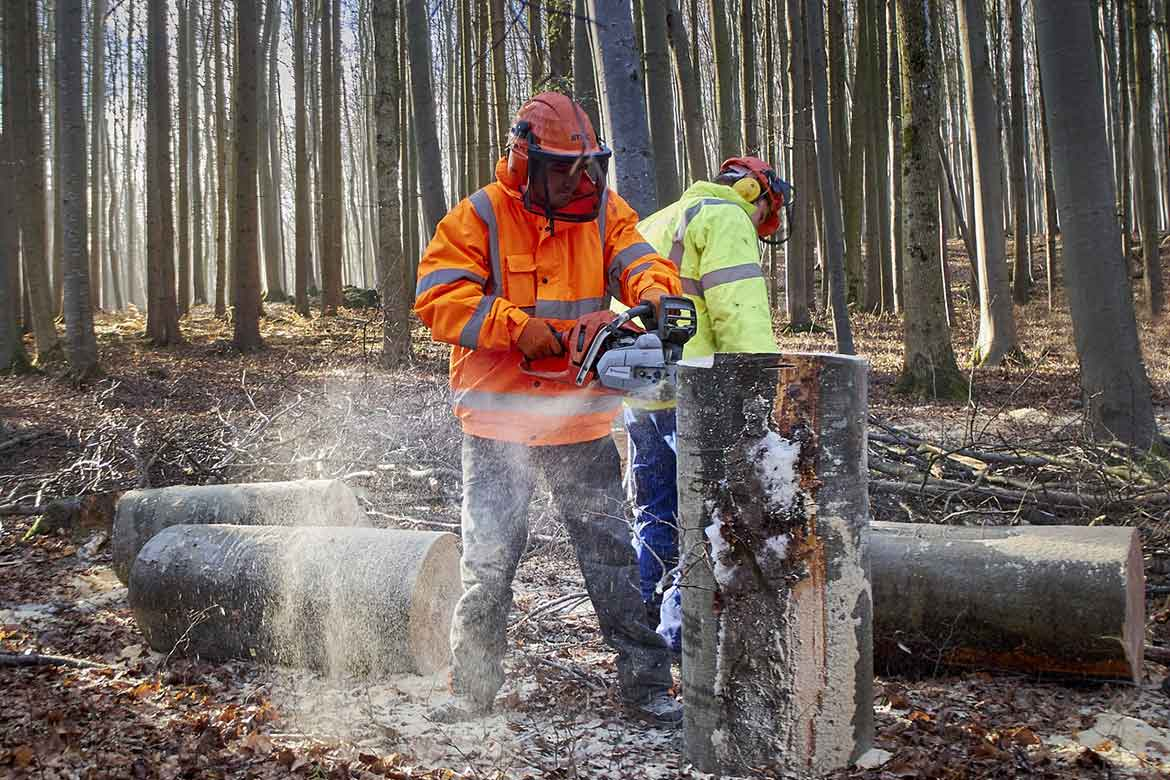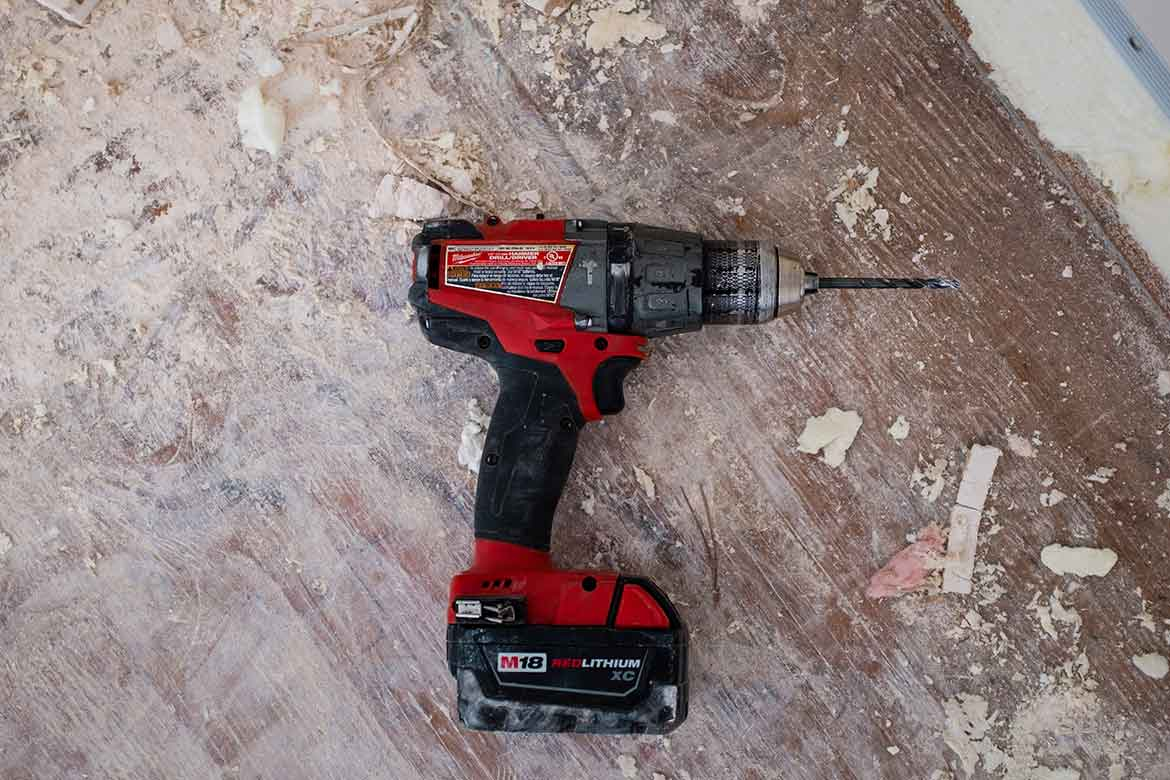24th September, 2015

# How To Measure Vibration Exposure To The EAV

The Control of Vibration at Work Regulations enforce an exposure value for vibration at work that you must be aware of, the Exposure Action Value (EAV). Where an employee is exposed to vibration levels over and above this value, you should look for ways to control vibration.In our last post we looked at how to calculate vibration exposure to the ELV. The Exposure Limit Value (ELV) is an important value that must not be exceeded, but The Control of Vibration at Work Regulations enforce another exposure value for vibration at work that you must be aware of, the Exposure Action Value (EAV).

The Control of Vibration at Work Regulations set an exposure action value (EAV) of 2.5m/s²A(8).

Where an employee is exposed to vibration levels over and above this value, you should look for ways to control vibration. This could be done through elimination or reduction of exposure, or exposure for shorter periods.

But how do we calculate when the EAV is reached.

Well, the EAV (2.5) is half the ELV (5), so would be reached in the half the time, right?

Wrong!

But you can follow a similar principle to the steps we followed in the previous blog post to calculate the ELV, to calculate the EAV.

Or you can cheat and use our free havs calculator (we won't tell anyone!).

We will again be using a points-based system, as this is the easiest way to calculate exposure once we take into account that operatives may be using various vibrating tools throughout a day.

So we were allowed 400 points a day to reach the ELV (the limit value - this is the maximum exposure).

We are allowed 100 points a day before we reach the action value (after which action must be taken to control vibration and reduce the risks).

The exposure action trigger in a day in our points-based system is 100 points - which equals the EAV of 2.5m/s²A(8).

Now, we need to give each tool a number of points per hour. This is how many points you use up for each hour on the tool.

(Output x Output) x 2 = Points

Ok, let's look at a 5m/s² tool.

(5 x 5) x 2 = 50

So that's 50 points per hour when using our 5m/s² tool.

We know we only have 100 points per day before we reach the EAV, so if we divide our 100 points by the points per hour, this tells us how long we can use the tool for.

100 / 50 = 2

2 hours. Once this time is up, you have reached the EAV, and you need to take action. Duties are to reduce the risks from vibration to the lowest level reasonably practicable and to reduce exposure to as low as is reasonably practicable if it is above the EAV.

Let's look at another example, this time with a higher vibration output. Let's look at a tool with an output of 10m/s².

(10 x 10) x 2 = 200

That's 200 points per hour when using our 10m/s² tool.

Note how the vibration output has doubled, but the points per hour has actually quadrupled.

Now we divide our 100 points by the points per hour, this tells us how long we can use the tool for.

100 / 200 = 0.5

0.5 hours (half an hour / 30 minutes). Once this time is up, you have reached the EAV, and you need to take action.

Remember, there is another limit (the Exposure Limit Value or ELV), which must not be exceeded... we talk about calculating that value here.

This article was written by Emma at HASpod. Emma has over 10 years experience in health and safety and BSc (Hons) Construction Management. She is NEBOSH qualified and Tech IOSH.

### HAVS Awareness Course

Learn how to control vibration, avoid hand arm vibration syndrome, and stay within the legal limits. Find out more and get your certificate.

## Recent posts like this...### How To Calculate Vibration Exposure To The ELV

The exposure limit value (ELV) under the Control of Vibration at Work Regulations is a value you must not exceed. But how do we calculate this limit? Calculating the ELV in real-life situations is not always straight forward, but using a points-based system, you can keep track of exposure levels.### Understanding Your HAVS Trigger Times

HAVS trigger times are how long your hands and arms are exposed to vibration levels. Trigger times can often get overestimated because they are not always fully understood. In this post, we look at what trigger times are and how to measure them when assessing vibration exposure.### HAVS Exposure Limits (EAV, ELV And What They Mean)

There are two exposure limits when it comes to HAVS, and these are legal limits as to the amount of vibration you can be in contact with on a daily basis. These limits are defined in the Control of Vibration at Work Regulations, the exposure limit value (ELV) and the exposure action value (EAV).